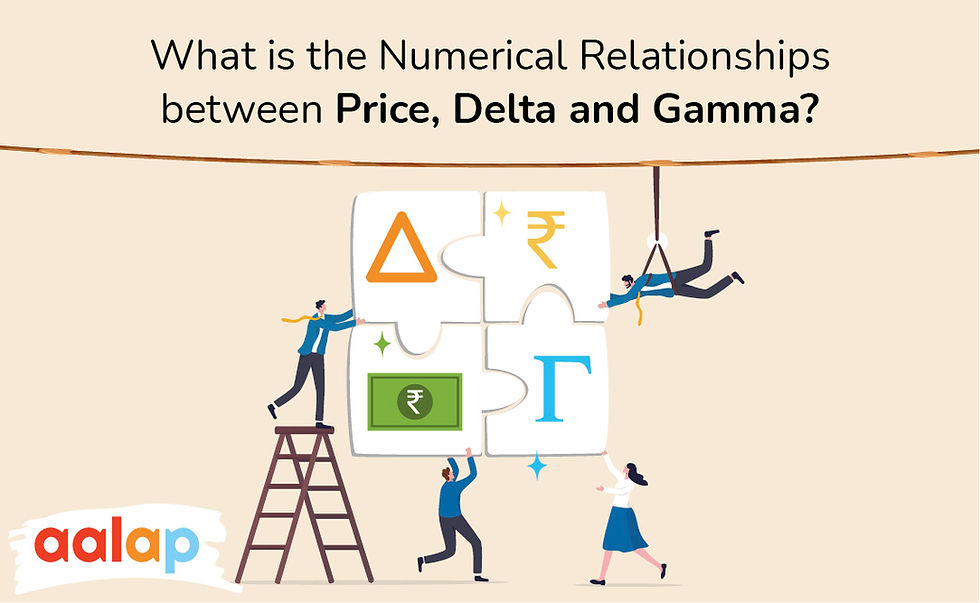top of page

# What is the Numerical Relationships between Price, Delta, and GammaTo understand the relationship between Underlying Price, Gamma Options, and Delta Options, you must be aware of these words' actual differences and relationships. These are frequently used terms for almost all options. The Price of Underlying has a different meaning than the Market Price or the commonly used Strike Price.

## What is the Underlying Price?

For every option, the strike price is already fixed and defined. This price will be used when the owner wants to exercise the option. On the other hand, the market price is a price you pay when you wish to buy the option, and in the same way, this is the price you get when you intend to sell the option.

The Underlying Price is an important factor that helps determine the underlying assets' derivatives, warrants, and securities price. This happens with a simultaneous change of the underlying asset price, which ultimately results in simultaneous changes in the derivatives assets' price associated with it.

The derivatives are normally associated with the value and price of the other asset. The underlying stock price goes up, and the derivative market price declines.

## What is “Delta Options”?

The delta is considered a derivative of the option price and is often known as the first-order

derivative. The second-order derivative is known as Gamma, a derivative of the delta. The underlying price is moved by the change rate in delta for every such 1 point.

Delta Options are for the risk measurement, and on the other hand, Gamma Options are important to measure the directional risk changes. Gamma Options is very useful in interpreting the potential movement of the delta that the changes in the underlying price may cause.

A Trader can access the changes happening in his position risk based on the value of Gamma. It is easy to express Gamma using the below simple equation.

• Change in Delta or Delta Change = Gamma * Change in the underlying price

Here you need to understand,

• Changes in the underlying prices = new underlying price - old underlying price.

To calculate the New Delta value, use the following equation.

• New Delta Value = Old Delta Value + Change in the Delta Value

To understand this Numerical Relationship between Price, Delta and Gamma, let us take an example.

Let us assume that a trending stock price is Rs. 100. The strike price of the ATM Call option is Rs. 100. So let us think that the Delta Option is 0.5, whereas the Gamma Options is 0.02. The price of the option is Rs. 6.

If the stock price increases and becomes Rs. 105 within a short time, we can easily calculate the new option price. If the older option price is Rs. 6, we need to add a delta that is 0.5 and add the Rs. 5 underlying price change. So, overall it becomes Rs. 8.5.

The option has moved the ITM, and now the underlying price has changed. So the delta is also going to change. Now here is what you need to do to calculate the new delta.

First, take the Old Data, which is 0.5, and then add Gamma 0.02. Then it would help if you multiplied it by the Change in the underlying price. So it will turn out to be about 0.6. Now, the 5-point rise in the new Underlying will be 0.6, and the new delta will be around 5. Now you will see that the options are now moved to ITM. Delta, on the other hand, moved higher than 0.5

You should also anticipate what could have happened to the stock price like it would go down somewhere like Rs. 95. So, here you need to assume that the option price may have gone down to Rs. 3.5.

The changes happening in the underlying would cause the Change of Delta value. If you wish to calculate this Change, then here is the way,

0.02 Gamma will lead to (-0.5) multiplication, so 0.02 * (-.5). So the answer will be -0.1. Now the new obtained Delta value would be 0.5 + (-0.1) = 0.4.

Now the options will move towards the state of OTM. And here, the delta will go down by 0.5.

## Gamma and Delta Relationship

Gamma experiences predictable changes. Delta will increase when an option moves farther into the money until it matches the underlying dollar for dollar, but delta can never be more significant than 1 or less than -1. When delta is close to 1 or -1, Gamma is close to 0 since delta does not fluctuate much with the underlying price.

Gamma and delta are maximal when an option is at the money or when the strike price equals the underlying price. The delta change is highest for options at the money and decreases as the option moves farther into or out of the money.

As the option moves further out of the money, both gamma and delta move towards zero.